# javascript实现二叉搜索树

### 二叉树的非递归先序、中序、后续遍历算法。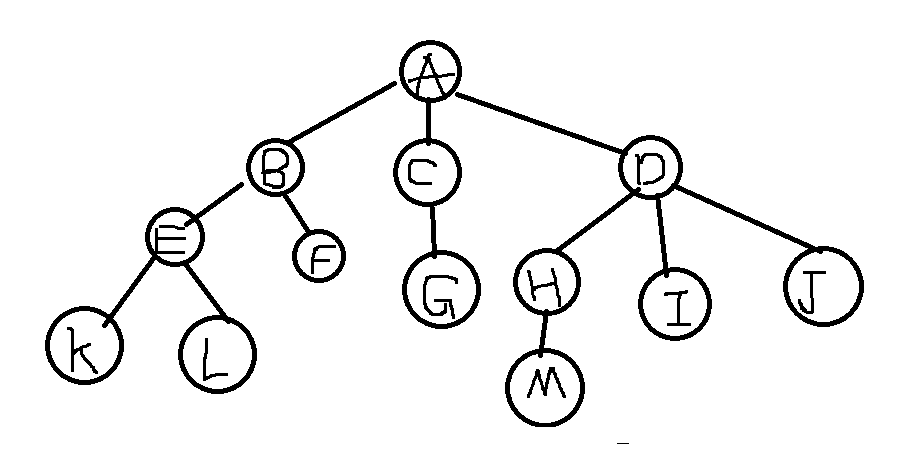## 树的基本术语：

1）每个结点最多只有两棵子树，即二叉树中的节点的度只能为0、1、2

2) 子树有左右之分，不能颠倒。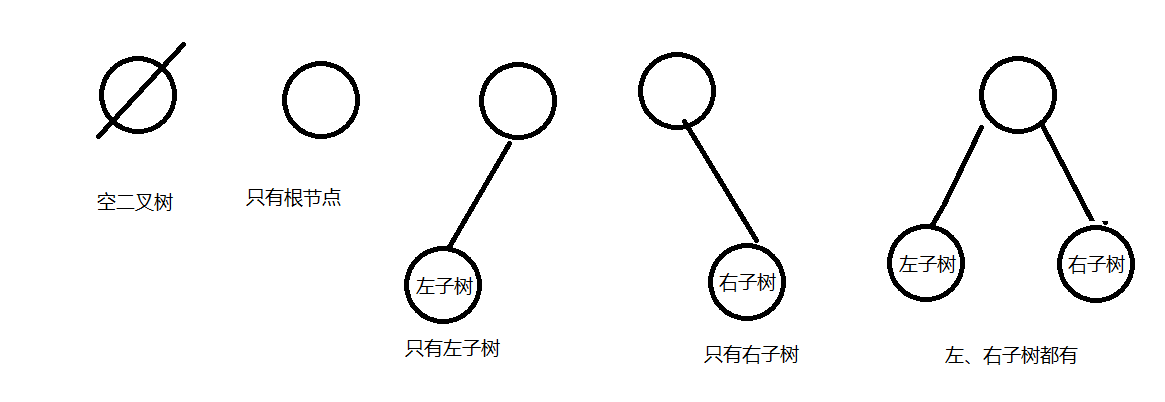## 构造一个二叉树的节点存储结构

1 // 树的结构
2 class BTNode {
3   constructor() {
4     this.key = key;
5     this.lchild = null;
6     this.rchild = null;
7   }
8 }

## 实现一个二叉搜索树 / 二叉排序树

1) 若它的左子树不空，则左子树上的所有关键字的值均小于根关键字的值。

2）若它的右子树不空，则右子树上所有关键字的值均大于根关键字的值。

3）左右子树又是一棵二叉排序树。

## 举个例子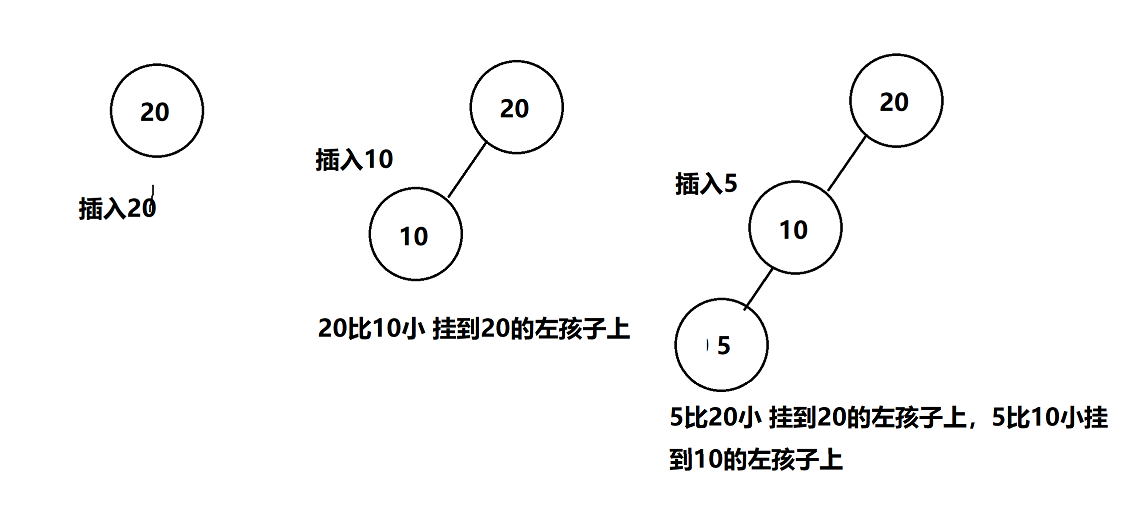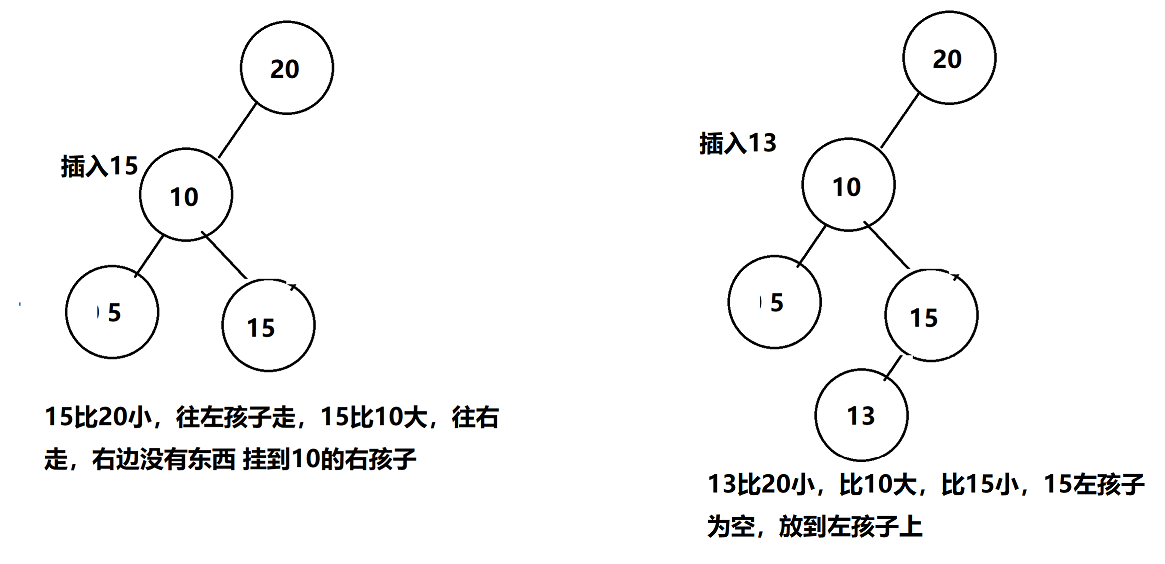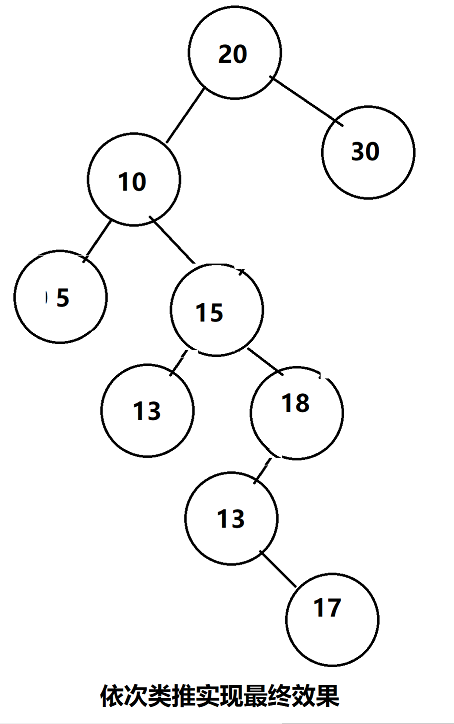那么用代码如何实现呢？

 1 let BST = (function () {
2
3   let ROOT = Symbol();
4
5   // 节点结构
6   let BTNode = class {
7     constructor(key) {
8       this.key = key;
9       this.lchild = null;
10       this.rchild = null;
11     }
12   }
13
14   // 递归插入节点
15   let recursionInsert = function (root, node) {
16     if (node.key < root.key) {
17       if (root.lchild) {
18         recursionInsert(root.lchild, node);
19       } else {
20         root.lchild = node;
21       }
22     } else {
23       if (root.rchild) {
24         recursionInsert(root.rchild, node);
25       } else {
26         root.rchild = node;
27       }
28     }
29   }
30
31   // 二叉搜索树类
32   return class {
33     constructor() {
34       this[ROOT] = null;
35     }
36
37     // 插入
38     insert(key) {
39       let node = new BTNode(key);
40       let root = this[ROOT];
41       if (!root) {
42         this[ROOT] = node;
43         return;
44       }
45       // 递归插入
46       recursionInsert(root, node);
47     }
48   }
49
50 })();
51
52
53 let bst = new BST();
54
55
56 bst.insert(20);
57 bst.insert(10);
58 bst.insert(5);
59 bst.insert(15);
60 bst.insert(13);
61 bst.insert(18);
62 bst.insert(17);
63 bst.insert(30);
64
65 console.log(bst);

在浏览器中一层一层的展开看看是否和我们的一样。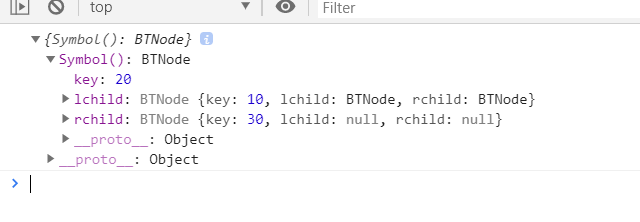## 二叉树的遍历算法

  1 let BST = (function () {
2
3   let ROOT = Symbol();
4
5   // 节点结构
6   let BTNode = class {
7     constructor(key) {
8       this.key = key;
9       this.lchild = null;
10       this.rchild = null;
11     }
12   }
13
14   // 递归插入节点
15   let recursionInsert = function (root, node) {
16     if (node.key < root.key) {
17       if (root.lchild) {
18         recursionInsert(root.lchild, node);
19       } else {
20         root.lchild = node;
21       }
22     } else {
23       if (root.rchild) {
24         recursionInsert(root.rchild, node);
25       } else {
26         root.rchild = node;
27       }
28     }
29   };
30
31   // 用于中序遍历二叉树的方法
32   let inorderTraversal = function (root, arr) {
33     if (!root) return;
34     inorderTraversal(root.lchild, arr);
35     arr.push(root.key);
36     inorderTraversal(root.rchild, arr);
37   };
38
39   // 用于先序遍历的递归函数
40   let preOrderTraversal = function (root, arr) {
41     if (!root) return;
42     arr.push(root.key);
43     preOrderTraversal(root.lchild, arr);
44     preOrderTraversal(root.rchild, arr);
45   };
46
47   // 用于后续遍历的递归函数
48   let lastOrderTraversal = function (root, arr) {
49     if (!root) return;
50     lastOrderTraversal(root.lchild, arr);
51     lastOrderTraversal(root.rchild, arr);
52     arr.push(root.key);
53   };
54
55   // 二叉搜索树类
56   return class {
57     constructor() {
58       this[ROOT] = null;
59     }
60
61     // 插入
62     insert(key) {
63       let node = new BTNode(key);
64       let root = this[ROOT];
65       if (!root) {
66         this[ROOT] = node;
67         return;
68       }
69       // 递归插入
70       recursionInsert(root, node);
71     }
72
73
74     // 中序遍历二叉树
75     inorderTraversal() {
76       let arr = [];
77       inorderTraversal(this[ROOT], arr);
78       return arr;
79     }
80
81     // 先序遍历二叉树
82     preOrderTraversal() {
83       let arr = [];
84       preOrderTraversal(this[ROOT], arr);
85       return arr;
86     }
87
88     // 后续遍历
89     lastOrderTraversal() {
90       let arr = [];
91       lastOrderTraversal(this[ROOT], arr);
92       return arr;
93     }
94   }
95
96 })();
97
98
99 let bst = new BST();
100
101 bst.insert(20);
102 bst.insert(15);
103 bst.insert(7);
104 bst.insert(40);
105 bst.insert(30);
106 bst.insert(45);
107 bst.insert(50);
108
109
110 console.log(bst);
111
112
113 let a = bst.inorderTraversal();
114 let b = bst.preOrderTraversal();
115 let c = bst.lastOrderTraversal();
116
117 console.log(a);
118 console.log(b);
119 console.log(c);

// 广度优先遍历
// 初始化用于广度优先遍历的队列
let queue = new Queue();
console.log('根节点', this[ROOT]);

let arr = [];
let root = this[ROOT];
if (!root) return;

queue.enqueue(root);

while (queue.size()) {
let queueFirst = queue.dequeue();
arr.push(queueFirst.key);
queueFirst.lchild && queue.enqueue(queueFirst.lchild);
queueFirst.rchild && queue.enqueue(queueFirst.rchild);
}

return arr;
}

## 二叉树遍历算法的改进

### 栈数据结构，满足后进先出的规则用来辅佐我们遍历

// 栈结构 用来辅助非递归遍历
class Stack {
constructor() {
this.items = [];
}
push(data) {
this.items.push(data);
}
pop() {
return this.items.pop();
}
peek() {
return this.items[this.items.length - 1];
}
size() {
return this.items.length;
}
}

### 非递归的先序遍历

preOrderTraversal() {
console.log('先序遍历');
let root = this[ROOT];
// 初始化辅助遍历存储的栈
let stack = new Stack();

let arr = []; // 用于存储先序遍历的顺序

stack.push(root);

// 如果栈不为空 则一直走
while (stack.size()) {
let stackTop = stack.pop();

// 访问栈顶元素
arr.push(stackTop.key);
stackTop.rchild && stack.push(stackTop.rchild);
stackTop.lchild && stack.push(stackTop.lchild);
}

return arr;
}

### 非递归的中序排序

// 中序遍历二叉树
inorderTraversal() {
// 初始化用于辅助排序的栈
let stack = new Stack;

let p = null; // 用于指向当前遍历到的元素
let arr = []; // 用户记录排序的顺序
p = this[ROOT];

while (stack.size() || p !== null) {
while (p !== null) {
stack.push(p);
p = p.lchild;
}

// 如果栈不为空 出栈
if (stack.size()) {
p = stack.pop();
arr.push(p.key);
p = p.rchild;
}
}
return arr;
}

### 非递归的后序排序

// 中序遍历二叉树
inorderTraversal() {
// 初始化用于辅助排序的栈
let stack = new Stack;

let p = null; // 用于指向当前遍历到的元素
let arr = []; // 用户记录排序的顺序
p = this[ROOT];

while (stack.size() || p !== null) {
while (p !== null) {
stack.push(p);
p = p.lchild;
}

// 如果栈不为空 出栈
if (stack.size()) {
p = stack.pop();
arr.push(p.key);
p = p.rchild;
}
}
return arr;
}

想一下，如果我们的插入顺序第一个数非常大，然后后面的数字都是越来越小的会有什么问题产生呢？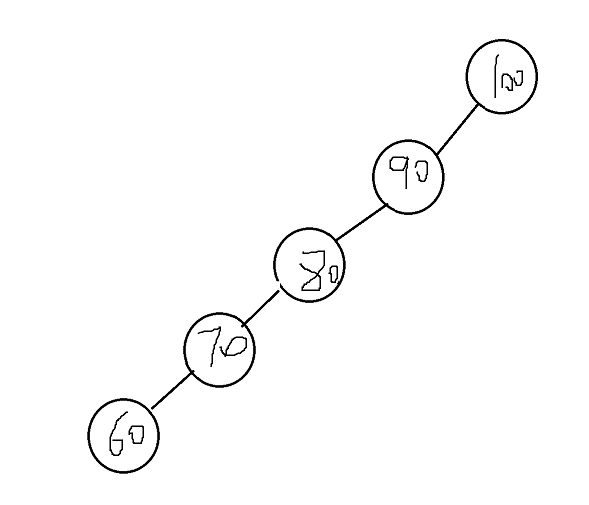posted @ 2019-09-18 18:13  一步一步向上爬  阅读(305)  评论(0编辑  收藏